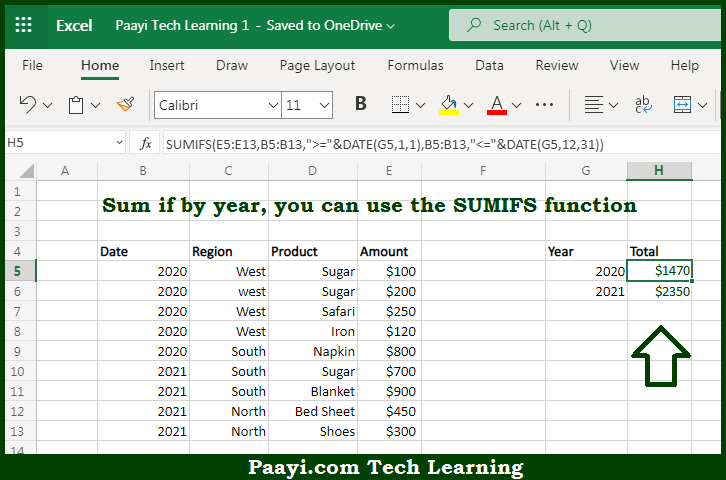# Learn How to SUM If By Year in Microsoft Excel

Written by | 0 Comments | 420 Views

In this article, you will learn how to SUM various things in Microsoft Excel using a single/combination(s) of functions. You will also know how to SUM If By Year and see the generic formula.

SUM If By Year in Microsoft Excel

The main purpose of this formula is to sum IF by year. Here we will learn how to sum if by year in the workbook in Microsoft Excel. That implies, with the help of a formula based on the SUMIFS function you can able to sum if by year. So, with the help of this formula, you can able sum it by year in the workbook in Microsoft Excel.

General Formula to SUM If By Year

=SUMIFS(sum_range,date,">="&DATE(year),date,"<="&DATE(year,12,31))

The Explanation for the SUM If By YearSo we know that with the help of the given formula above you can able sum IF by year. Here we will learn how to sum it by year in the workbook in Microsoft Excel. As we know that the SUMIFS function always uses the first argument in the range to sum that is "sum_range", and criteria are supplied as one or more range/criteria pairs. So, with the help of this formula, you can able to sum it by year in the workbook in Microsoft Excel.